Subsections

# Smooth and Inextensible Surface Reconstruction

Although the strategem of maximizing the sum of depthsdescribed in the previous section gives reasonable results, it is merely a heuristic, not based on any valid principle related to surface properties. We therefore consider next a new formulation based on the principle of surface inextensibility.

Let the surface be modelled as a function, mapping the planar template to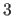-dimensional space. The inextensibility constraint is equivalent to saying that the map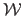must be everywhere a local isometry. This condition may be expressed in terms of its Jacobian. Letbe the Jacobian matrix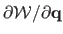evaluated at the point. The mapis an isometry atif the columns of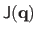are orthonormal. This local isometry can be enforced for the whole surface with the following least-squares constraint: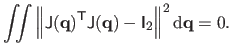(7.7)

In practice, we consider a discretization of the quantity in equation (7.7), namely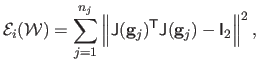(7.8)

where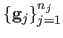is a set of 2D points in the template image space taken on a fine and regular grid (for instance, a grid of size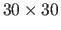). This termmeasures the departure from inextensibility of the surface.

Our minimization problem is then to minimize this quantity, over all possible surfaces, subject to the projection constraints, namely that pointprojects to (or near to) the image point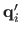, for all.

## Parametric Surface Model

The problem just described involves a minimization over all possible surfaces. Instead of considering this as a variational problem over all possible surfaces, we consider a parametrized family of surfaces. For this purpose, we chose Free-Form Deformations (FFD) (162) based on uniform cubic B-splines (61). All the details on this parametric model have been given in section 2.3.2. Here, we just precise the notation used in this chapter. Letbe the parametric FFD, parametrized by a family of 3D points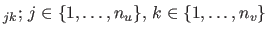, which act as attractors' for the surface.

For a pointin the template, the surface point is explicitly given as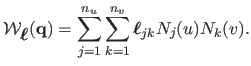(7.9)

The functionsare the B-spline basis functions (61) which are polynomials of degree. If point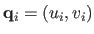is fixed and known then the surface point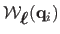is expressed as a linear combination of the points, and hence can be written in the form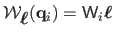, where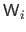is a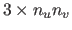matrix depending only on the point, andis the vector obtained by concatenating all the points. Thus, the 3D point is a linear expression in terms of the parameter vector. Since the polynomialsand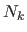depend only on a local set of the attractor points, the matrixis sparse, which is important for computational efficiency.

## Surface Reconstruction as a Least-Squares Problem

By replacing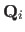byin equation (7.6) we may arrive at a constraint: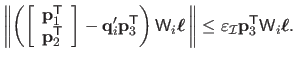(7.10)

We may then formulate the optimization problem as minimizing the inextensibility costgiven in equation (7.8) over all choices of parameters, subject to constraints equation (7.10). The constraints are SOCP constraints, but the cost function equation (7.8) is of higher degree in the parameters. To avoid the difficulties of constrained non-linear optimization, we choose a different course, by including the reprojection error into the cost function, leading to an unconstrained problem.

To simplify the formulation of the reprojection error, we introduce the depths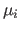as subsidiary variables, for reasons that become evident below. This is not strictly necessary, but reduces the degree of the reprojection-error term. The minimization problem now takes the form: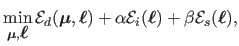(7.11)

where,,are the data (reprojection error), inextensibilty, and smoothing terms respectively. The data term ensures the consistency of the point correspondences with the reconstructed surface.forces the inextensibility of the surface.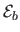promotes smooth surface in order to cope with, for instance, lack of data. The relative influence of these three terms are controlled with the weights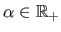and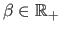. Note that the choice ofandis generally not critical.

The inextensibility term has been described previously. We now describe the two other terms in equation (7.11).

#### Data term.

Replacingbyin equation (7.5) gives an expression for the reprojection error associated with some point. However, the resulting expression is non-linear with respect to the parameters. We thus prefer a linear data term expressed in terms of 3D errors', which is the reason why we introduced the depths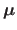of the data points in the optimization problem. The data term is then defined by: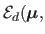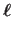(7.12)

which measures the distance between the point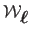on the surface and the point at depthalong the ray defined by.

#### Smoothing term.

In some cases, the point correspondences and the hypothesis of an inextensible surface are not sufficient. For instance, imagine that there is no point correspondence in a corner of the surface. In this case, there is nothing that indicates how the surface should behave. The corners of the surface can bend freely as long as they do not extend or shrink (like the corners of a piece of paper). To overcome this difficulty, we can add a third term (the smoothing term) in our cost function that favours non-bending surfaces. Note that usually, such terms are used to compensate for the undesirable effects of under-fitting and over-fitting. Doing so is usually a problem because it requires one to determine a correct value for the weight associated to the smoothing term (valuein equation (7.11)). This is a sensible and critical way of balancing the effective complexity of the surface against the complexity of the data. Here, we do not have to care too much. Indeed, the complexity of the surface is limited by the fact that it is inextensible. Any small value (but big enough to be not negligible, for instance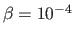) is thus suitable for the weight of the smoothing term. We define our smoothing term using the bending energy: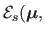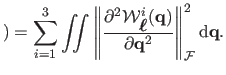(7.13)

where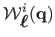is the-th coordinate of the point, and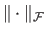is the Frobenius norm of the Hessian matrix. With FFD, there exists a simple and linear closed-form expression for the bending energy: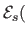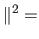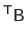(7.14)

whereis a symmetric, positive, and semi-definite matrix which can be easily computed from the second derivatives of the B-spline basis functions.

### Initial solution.

The problem of equation (7.11) is a non-linear least-squares minimization problem typically solved using an iterative scheme such as Levenberg-Marquardt. Such an algorithm requires a correct initial solution. We used an FFD surface fitted to the 3D points reconstructed with one of the point-wise methods presented in section 7.3. Subsequently, since we use a surface model which is linear with respect to its parameters, the initial parameterscan be found by solving the least-squares problem: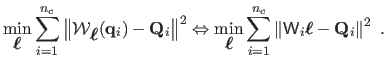(7.15)

An alternative is to modify the problem (7.6), expressingin terms of the required parameters, according to. Then one may solve fordirectly using SOCP. If necessary, the linear smoothing term of equation (7.13) can be included in equation (7.15).

Contributions to Parametric Image Registration and 3D Surface Reconstruction (Ph.D. dissertation, November 2010) - Florent Brunet
Webpage generated on July 2011